April 2020 was warmer than average (10.04°C versus an average of 8.56), drier than average (14.40 mm versus an average total of 36.84) and calmer than average (a wind run of 2552 miles versus an average of 2817). The maximum temperature in the month was 22.3°C recorded at 15:12 on the 11th, the highest temperature since 27.8°C was recorded on August 27th 2019. The minimum temperature was 0.0°C recorded at 08:22 on the 14th. The average temperature in the month was 10.0°C. The average daily high temperature was 15.4°C, and the average daily low temperature was 5.2°C. The warmest day [Read more]

February 2020 was warmer than average (5.67°C versus an average of 4.26), wetter than average (128.80 mm versus an average total of 35.20) and windier than average (a wind run of 5789 miles versus an average of 3076). The average temperature for the month was the third highest for February at 5.7°C. This was 1.3°C above the Februsry mean. The highest temperature in the month was 12.8°C at 12:32 on the 9th, with the lowest being -0.9°C at 08:09 on the 6th. This was the only day in the month when the temperature fell below zero. The average daily high [Read more]

January 2020 was warmer than average (6.04°C versus an average of 3.84), wetter than average (44.20 mm versus an average total of 42.34) and windier than average (a wind run of 3794 miles versus an average of 3318). The month was also the warmest January here, with the average temperature beating the previous high of 5.2°C set in 2016. The new decade started with unseasonally mild weather through the month with just four days of sub-zero temperatures from the 18th and 21st. The maximum temperature in the month was 12.8°C on the 14th at 15:53. The minimum temperature was -1.3°C [Read more]

December 2019 was warmer than average (5.25°C versus an average of 4.80), wetter than average (59.00 mm versus an average total of 50.82) and windier than average (a wind run of 3608 miles versus an average of 3328). The highest temperature in the month was 11.8°C recorded at 13:33 on the 6th, 0.4°C below the December mean.. The lowest temperature was -1.9°C recorded at 02:35 on the 2nd, 1.3°C below the mean and the lowest temperature of the Winter season so far. The monthly average temperature was 5.2°C, 0.4°C above the December average. The average daily high temperature was 7.8°C, [Read more]November 2019 was cooler than average (5.9°C versus an average of 6.9), wetter than average (142.20 mm versus an average total of 58.30) and calmer than average (a wind run of 2349 miles versus an average of 2797). The highest temperature in the month was 13.3°C recorded at 23:01 on the 1st. This equalled the lowest November maximum temperature set in 2012. The lowest temperature was -1.8°C recorded at 05:22 on the 19th, the lowest temperature of the Autumn season. At 06:21 on the 9th, the temperature fell to -0.7°C, the lowest temperature since -4.4°C was recorded on February 3rd. [Read more]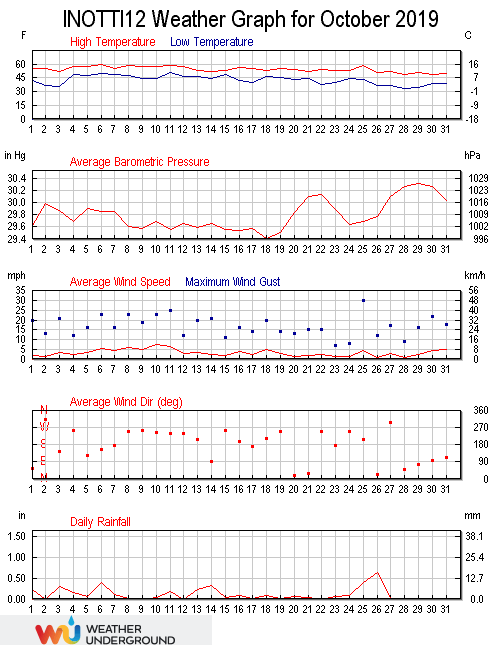October 2019 was cooler than average (9.1°C versus an average of 10.7°C), wetter than average (92.60 mm versus an average total of 47.62) and calmer than average (a wind run of 2435 miles versus an average of 2691). The highest temperature in the month was 15.2°C recorded at 13:51 on the 6th. The was the lowest October maximum ever recorded here. The lowest temperature was 0.8°C recorded at 06:04 on the 28th. The monthly average temperature was 9.1°C, only 2012’s 8.7°C was lower. The average daily hogh temperature 12.5°C, 1.4°C below the mean, with the average low temperature being 6.1°C, [Read more]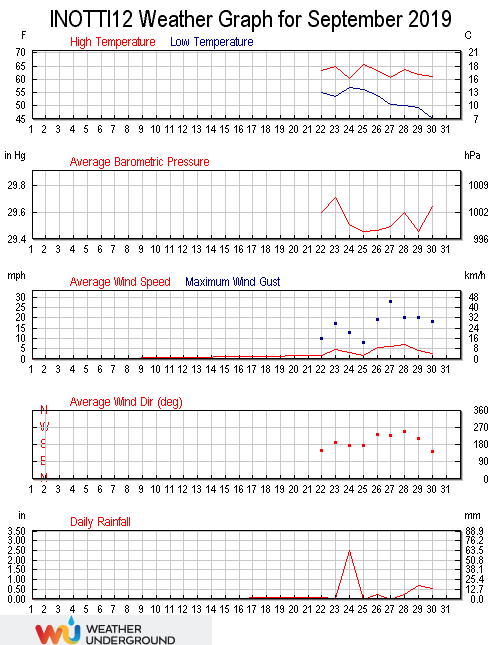September 2019 was equal to the average temperature at 13.4°C, wetter than average (104.20 mm versus an average total of 37.09) and windier than average (a wind run of 2388 miles versus an average of 2199). The highest temperature in the month was 22.6°C recorded at 16:03 on the 21st. The lowest temperature was 4.6°C recorded at 07:01 on the 8th and the monthly average was 13.4°C The average daily high temperature was 18.0°C, 0.3°C above the mean. The average daily low temperature was 9.6°C, the same as the mean. The warmest days were the 3rd and the 21st with [Read more]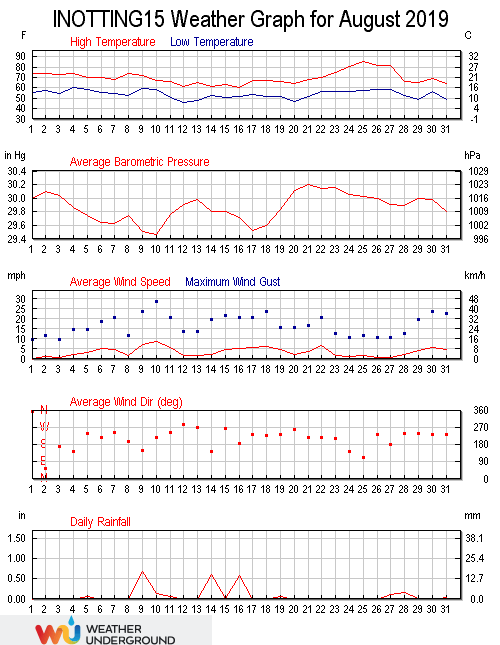August 2019 was warmer than average (16.7°C versus an average of 15.5), wetter than average (63.00 mm versus an average total of 49.91) and windier than average (a wind run of 2660 miles versus an average of 2272). The highest temperature in the month was 30.0°C recorded at 17:54 on the 25th, the second highest temperature of 2019, beaten only by the 34.1°C recorded on July 25th. This is only the sixth time that a temperature of 30.0°C has been recorded here. The minimum temperature was 8.1°C, recorded at 06:04 on the 12th. The average temperature in the month was [Read more]July 2019 was warmer than average (17.3°C versus an average of 16.4), wetter than average (82.80 mm versus an average total of 44.78) and calmer than average (a wind run of 1944 miles versus an average of 2044). The record temperature for this location was smashed in July 2019 when the thermometer reached 34.1°C at 18:37 on the 25th. This easily beat the previous record of 32.7°C set on July 1st 2015. This is only the fifth time that a temperature in excess of 30°C has been recorded here. The minimum temperature was 7.9°C recorded at 05:00 on the 2nd. [Read more]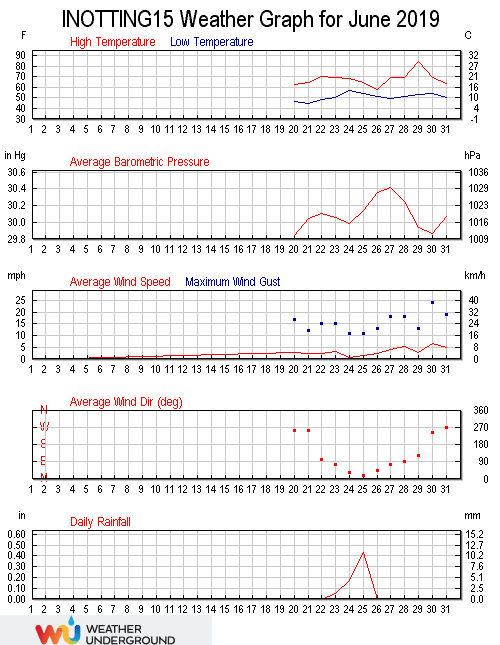June 2019 was cooler than average (13.7°C versus an average of 14.4), wetter than average (104.20 mm versus an average total of 52.56) and less windy than average (a wind run of 2240 miles versus an average of 2408). The highest temperature in the month was 29.4°C, the highest temperature of 2019 so far, and the highest temperature since 31.0°C was recorded on July 26th 2018. This was the second highest June temperature recorded here, beaten only by the 29.5°C recorded on June 19th 2017. The minimum temperature was 7.0°C recorded at 05:03 on the 16th. The average temperature in [Read more]May 2019 was cooler than average (11.0°C versus an average of 11.5), drier than average (23.40 mm versus an average total of 44.13) and calmer than average (a wind run of 2132 miles versus an average of 2724). The highest temperature in the month was 21.3°C recorded at 16:30 on the 30th, 1.9°C below the mean for the month. The minimum temperature was 0.4°C recorded at 05:01 on the 5th, equalling the lowest May temperature set on May 5th 2012. The average temperature in the month was 11.0°C, 0.5°C below the mean. The average daily high temperature was 15.6°C, 0.2°C [Read more]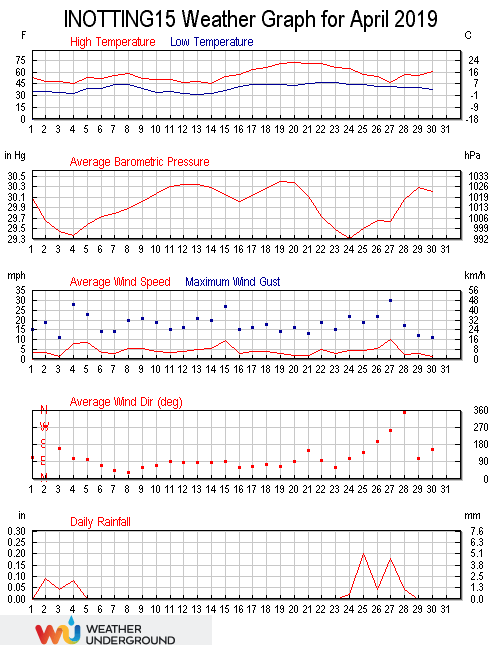April 2019 was warmer than average (8.6°C versus an average of 8.5°C), drier than average (17.60 mm versus an average total of 38.96) and windier than average (a wind run of 3094 miles versus an average of 2786). The month was very average with no records broken. The highest temperature in the month was at 17:04 on the 20th when the thermometer hit 22.2°C, the highest temperature of 2019 so far. The lowest temperature was -0.3°C at 06:15 on the 13th, the only time the temperature dropped below freezing in the month. The average temperature in the month was 8.6°C, [Read more]